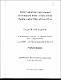## Power transmission lines transient electromagnetic fields-a study of scale modeling and the effects of ground loss2012-04-09
##### Authors
Gharagozloo, Pooya
##### Abstract
In this thesis, the problem of calculating power transmission line induced or radiated electromagnetic fíelds is studied by decomposing the line into a large number of snall segments, k¡own as Hertzian dipoles. Since the presence of lossy ground makes the electromagnetic fìeld distribution very different from that of the same dipole over perfectly conducting ground, different approaches should be followed for the lossy and lossless ground assumptions. The contribution of each dipole on the total electric and magnetic field is calculated using three analytical techniques. Two of these methods are frequency-domain solutions for the problem of dipole radiation above lossy ground, while the third method is a time-domain exact solution for the same problem based on the assumption of perfectly conducting ground. The theoretical background and extent of validity of each technique are reviewed in this thesis. Tlie results derived by applying each method are compared with those obtained using a commercial software package. The time-domain solution for the problem of power transmission line electromagnetic fields is obtained using Fourier and inverse Fourier transforms, rvhich enables us to obtain the electromagnetic waveforms associated with power system transients. The effect of different parameters such as conductivity and relative permittivity of the ground, the line{o-observation point distance, height, and sag of the transmission line is studied. Further, the impact of downscaling of transmission line on different electromagnetic parameters is analyzed, and the appropriate scale factor for each parameter is derived.
##### Keywords
power, transmission, electromagnetic, fields